AscendEX Margin Trading is a financial derivative instrument used for cash trading. While using the Margin Trading mode, AscendEX users can leverage their tradable asset to achieve a potential higher return on their investment. However, users must also understand and bear the risk of potential losses of Margin Trading.

Margin trading on AscendEX requires collateral to support its leverage mechanism, allowing users to borrow and repay at any point while margin trading. Users do not need to manually request to borrow or return. When users transfer their BTC, ETH, USDT, XRP, etc. assets to their “Margin Account”, all account balances can be used as collateral.

Trading on margin is the process by which users borrow funds to trade more digital assets than what they would normally be able to afford. Margin trading allows users to increase their buying power and potentially achieve a higher return. However, considering the digital asset’s high market volatility nature, users may also incur much greater losses with the use of leverage. Therefore, users should fully understand the risk of trading on margin before opening a margin account.

2.Margin Account
AscendEX margin trading requires a separate “Margin Account.”Users can transfer their assets from their Cash Account to their Margin Account as collateral for margin loan under the [My Asset] page.

3.Margin Loan
Upon successful transfer, the platform’s system will automatically apply the maximum leverage available based on the user’s “Margin Asset” balance. Users do not need to request a margin loan.

When the margin trading position exceeds the Margin Assets, the exceeding portion will represent the margin loan. The user’s margin trading position must stay within the specified Maximum Trading Power (limit).

For example:
A user’s order will be rejected when the total loan exceeds the account’s Maximum Borrowable Limit. The error code is displayed under Open Order/Order History section on trading page as ‘Not Enough Borrowable’. As a result, users will not be able to borrow more until they repay and reduce the outstanding loan under the Maximum Borrowable Limit.

4.Interests of Margin Loan
Users can only repay their loan with the token they borrowed. Interest on margin loans are calculated and updated on users’ accounts page every 8 hours at 8:00 UTC, 16:00 UTC and 24:00 UTC. Please note that any holding period less than 8 hours will be counted as an 8-hour period. No interest will be taken into account when borrowing and repaying actions are completed before the next margin loan gets updated.

Point Card Rules

5.Loan Repayment
AscendEX allows users to repay the loans by either transacting the assets from their Margin Account or transferring more assets from their Cash Account. Maximum trading power will be updated upon repayment.

Example:
When the user transfers 1 BTC to the Margin Account and the current leverage is 25 times, the Maximum Trading Power is 25 BTC.

Assuming at the price of 1 BTC = 10,000 USDT, buying additional 24 BTC with selling 240,000 USDT results in the loan (Borrowed Asset) of 240,000 USDT. The user can repay the loan plus interests by either making transfer from Cash Account or selling BTC.

Make a Transfer:
Users can transfer 240,000 USDT (plus interest incurred) from the Cash Account to repay the loan. Maximum trading power will increase accordingly.

Make a Transaction:
Users can sell 24 BTC (plus respective interest owed) through margin trading and the sales proceeds will automatically be deducted as loan repayment against borrowed assets. Maximum trading power will increase accordingly.

Note: Interest portion will be repaid prior to the loan’s principle.

6. Computation of Margin Requirement and Liquidation
In the Margin Trading, Initial Margin (“IM”) will be calculated first separately for user’s Borrowed Asset, user’s Asset and overall user accounts. Then the highest value of all will be used for the Effective Initial Margin (EIM) for the account. IM is converted to USDT value based on the current market price available.

EIM for the account= Maximum Value of (IM for all Borrowed Asset, IM for Total Asset, IM for the account)
IM for individual Borrowed Asset = (Borrowed Asset + Interest Owed)/ (Max Leverage for the Asset-1)
IM for all Borrowed Asset = Summation of (IM for individual Borrowed Asset)
IM for individual Asset = Asset / (Max Leverage for the Asset -1)
IM for Total Asset = Summation of all the (IM for individual Asset) * Loan Ratio
Loan Ratio = (Total Borrowed Asset + Total Interest Owed) / Total Asset
IM for the account = (Total Borrowed Asset + Total Interest Owed) / (Maximum Leverage for the account -1)

Example:
User’s position is shown as below: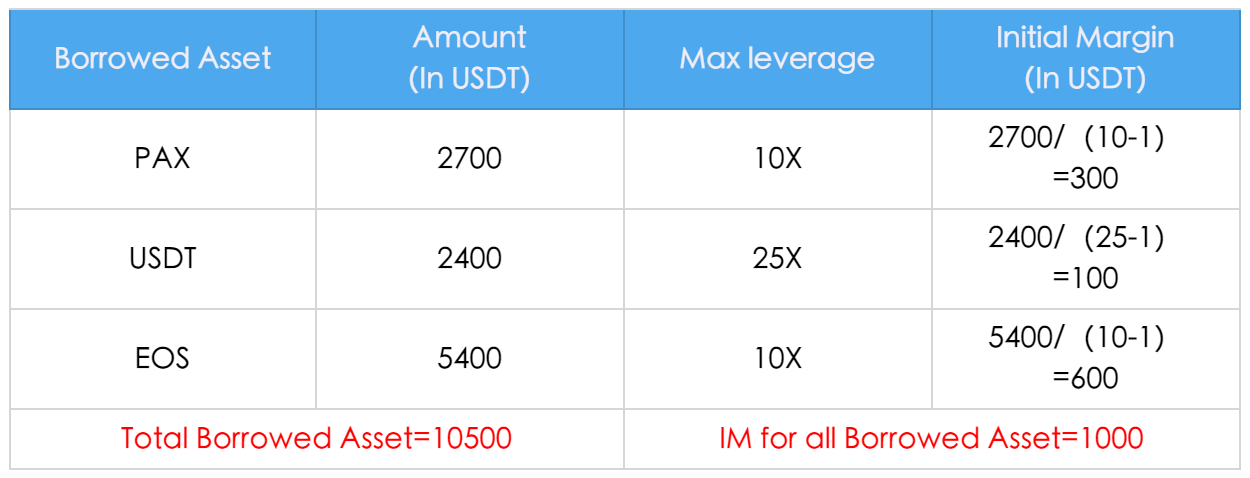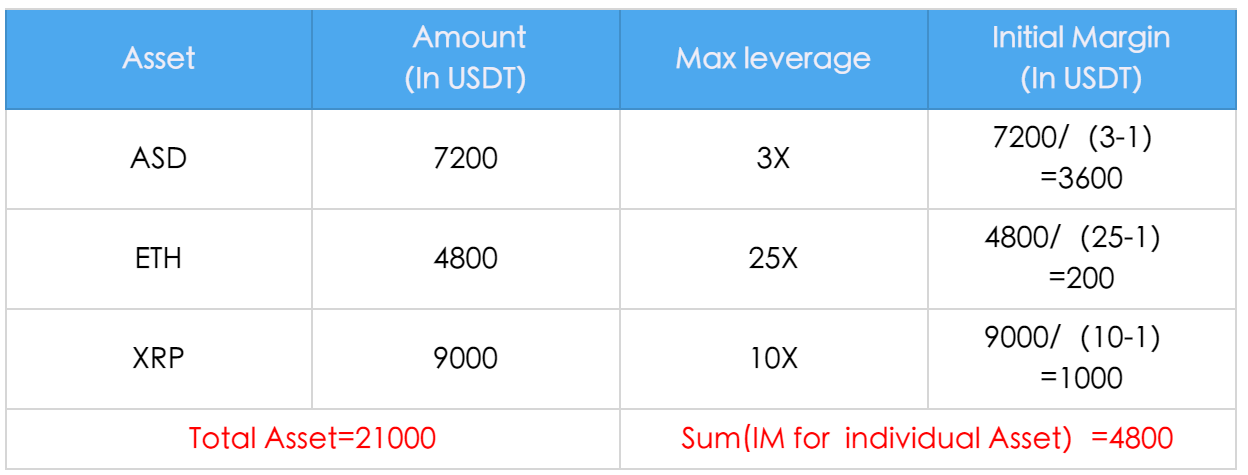Therefore, Effective Initial Margin for the account is calculated as follows: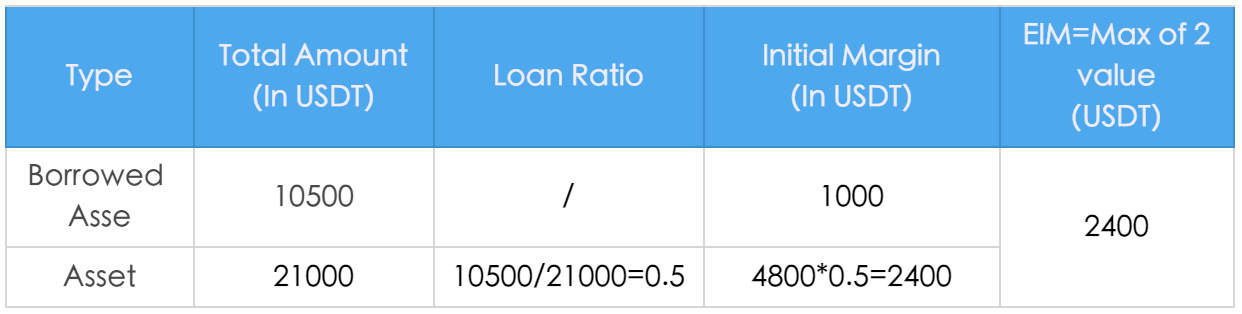Note:
For the purpose of illustration, Interest Owed is set at 0 in the example above.

When the current Net Asset of Margin Account is lower than the EIM, users cannot borrow more funds.

When the current Net Asset of Margin Account exceeds the EIM, users can place new orders. However, the system will calculate the impact of new order on the Net Asset of Margin Account based upon the order price. If the newly placed order will cause the new Net Asset of Margin Account to drop below the new EIM, the new order will be rejected.

Update of Effective Minimum Margin (EMM) for the account

Minimum Margin (MM) will first be calculated for user’s Borrowed Assets and Assets. The greater value of those two will be used for the Effective Minimum Margin for the account. MM is converted to USDT value based off the market price available.

EMM for the account = Maximum value of (MM for all Borrowed Asset, MM for Total Asset)

MM for individual Borrowed Asset = (Borrowed Asset + Interest Owed)/ (Max Leverage for the Asset*2 -1)

MM for all Borrowed Asset = Summation of (MM for individual Borrowed Asset)

MM for individual Asset = Asset / (Max Leverage for the Asset *2 -1)

MM for Total Asset = Summation of (MM for individual Asset) * Loan Ratio

Loan Ratio = (Total Borrowed Asset + Total Interest Owed) / Total Asset

An example of the user’s position is shown below: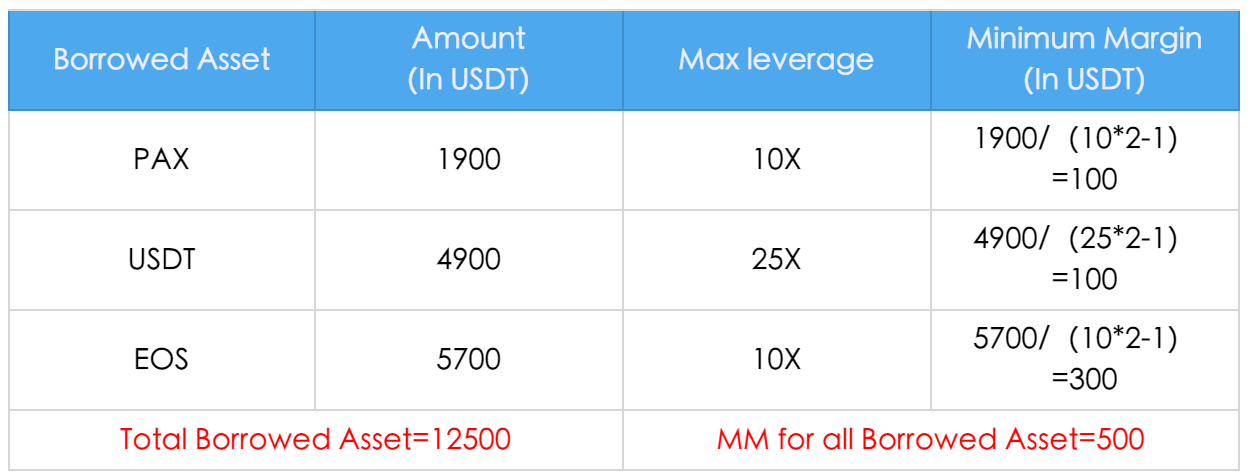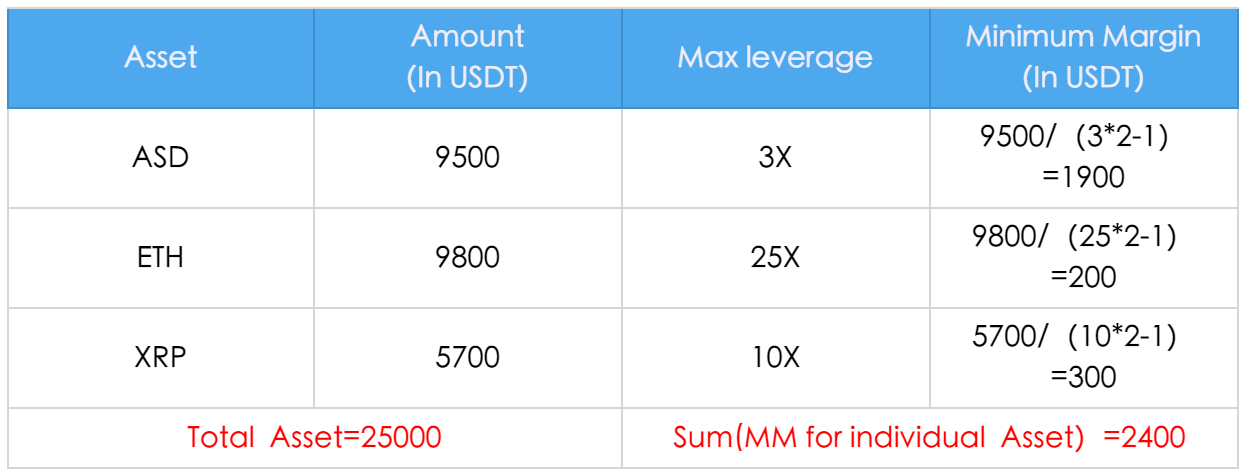Therefore, Effective Minimum Margin for the account is calculated as follows: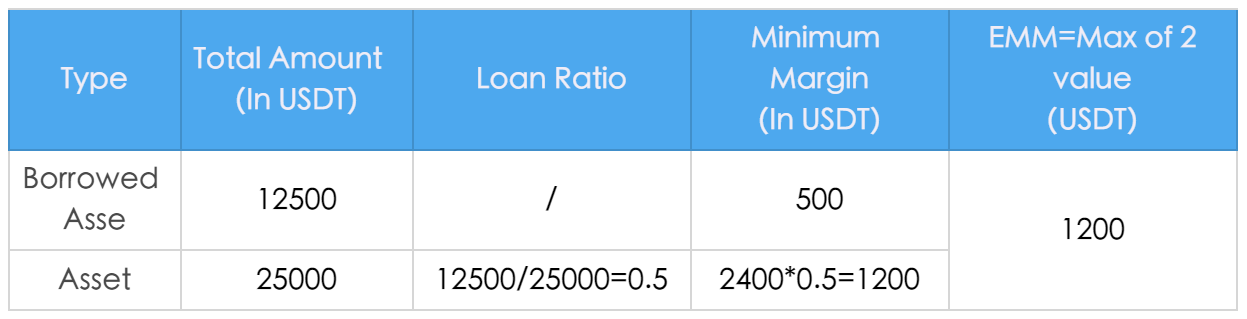Rules for Open Orders
Open order of margin trading will lead to the increase of Borrowed Asset even before the order execution. However, it will not affect the Net Asset.

Note:
For the purpose of illustration, Interest Owed is set as 0 in the example above.

Rules for Liquidation Process remain the same. When cushion rate reaches 100%, the user’s margin account will be subject to forced liquidation immediately.

Cushion rate = Net Asset of Margin Account / Effective Minimum Margin for the account.

The Calculation of Total Amount of Borrowed Assets and Assets

Under the Loan Summary section on the margin trading page, Balance and Loan Amount are displayed by asset.

Total Amount of Asset = Sum of Balance of all assets converted to the equivalent value of USDT based off the market price

Total Amount of Borrowed Asset = Sum of Loan Amount for all assets converted to the equivalent value of USDT based off the market price.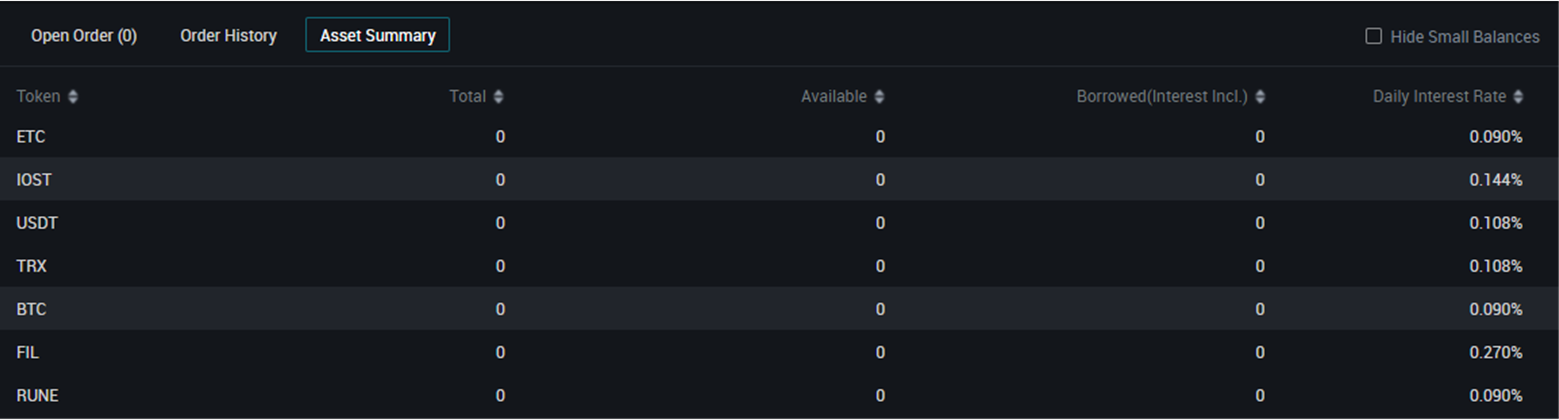Current Margin Ratio = Total Asset / Net Asset (which is Total Asset – Borrowed Asset – Interest Owed)

Cushion = Net Asset/Min Margin Req.

Margin Call: When cushion reaches 120%, the user will receive a margin call via email.

Liquidation: When cushion reaches 100%, the user’s margin account may be subject to liquidation.

7.Liquidation Process

Reference Price
In order to mitigate price deviation due to market volatility, AscendEX uses composite reference price for the calculation of margin requirement and forced liquidation.  The reference price is computed by taking an average last trade price from the following five exchanges (upon availability at the time of computation)- AscendEX, Binance, Huobi, OKEx and Poloniex, and removing the highest and lowest price.

AscendEX reserves the right of updating pricing sources without notice.

Process Overview

1. When the cushion of the margin account reaches 1.0, forced liquidation will be executed by the system, namely the forced liquidation position will be executed in the secondary market;
2. If the cushion of the margin account reaches 0.7 during forced liquidation or the cushion is still below 1.0 after the forced liquidation position is executed, the position will be sold to the BLP;
3. All functions will be automatically resumed for the margin account after the position is sold to the BLP and executed, namely the balance of the account is not negative.

8.Fund transfer
When a user's Net Assets are greater than 1.5 times that of the Initial Margin, the user can transfer assets from their Margin Account to their Cash Account as long as the Net Asset remains higher or equal to 1.5 times of the Initial Margin.

9.Risk Reminder
While margin trading can boost buying power for a higher profit potential with the use of financial leverage, it can also amplify the trading loss if the price moves against the user. Therefore, user should limit the usage of high margin trading in order to mitigate risk of liquidation and even greater financial loss.

10.Case Scenarios
How to trade on margin when the price goes up? Here is an example of BTC/USDT with 3x leverage.
If you expect that BTC price would go up from 10,000 USDT to 20,000 USDT, you can borrow maximum of 20,000 USDT from AscendEX with 10,000 USDT capital. At the price of 1 BTC = 10,000 USDT, you can buy 25 BTC and then sell them when the price doubles. In this case, your profit would be:

25*20,000 – 10,000 (Capital Margin) – 240,000 (Loan) = 250,000 USDT

Without the margin, you would only have realized PL gain of 10,000 USDT. In comparison, margin trading with 25x leverage amplifies the profit by 25 times.

How to trade on margin when the price drops? Here is an example of BTC/USDT with 3x leverage:

If you expect that BTC price would drop from 20,000 USDT to 10,000 USDT, you can borrow maximum of 24 BTC from AscendEX with 1BTC capital. At the price of 1 BTC = 20,000 USDT, you can sell 25 BTC and then buy them back when the price drops by 50%. In this case, your profit would be:

25*20,000 – 25*10,000= 250,000 USDT

Without the ability to trade on margin, you would not able to short the token in anticipation of falling price.